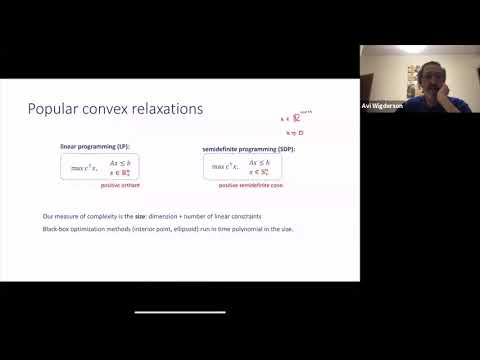## Approximating Max Cut with Subexponential Linear Programs

March 29, 2021
IAS
Presenters: Tselil Schramm

Watch Video

#### Abstract

In the max cut problem, we are given an n-vertex graph and we are asked what is the maximum fraction of edges "cut" by any partition of the vertices? This problem can be reformulated as an optimization problem over the cut polytope, which has exponentially many vertices and facets. A classical result of Goemans and Williamson proves that we can approximate the max cut value of a graph within a factor of 0.878, by instead optimizing over a spectrahedral relaxation of polynomial complexity. The theory-of-approximation orthodoxy (for good reason) predicts that polytope relaxations are far inferior, and small polytopes cannot hope to give non-trivial approximation to max cut. In this talk, I will describe a result which defies this prediction: we show that polytopes with subexponentially many facets *can* give nontrivial approximations to the max cut problem, as well as other combinatorial optimization problems. This is in surprising contrast to optimization problems over the reals, for which an exponential separation between spectrahedra and polytopes is known.